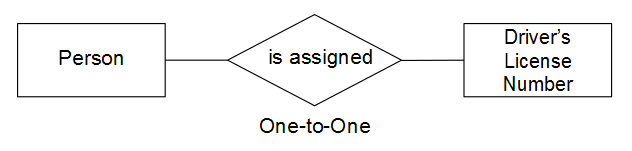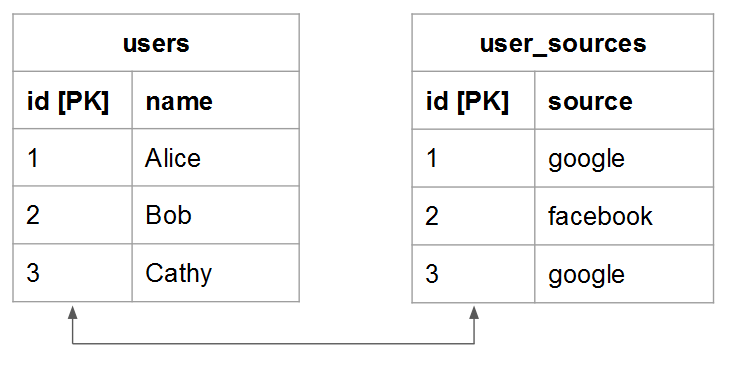# Understand and Evaluate Table Relationships

When assessing the relationship between two given tables, you need to understand how many possible occurrences in one table could belong to an entity in another, and vice versa. For example, Let us use a `users` table and an `orders` table. In this case, you want to know how many orders a given user has placed and how many possible users an order could belong to.

Understanding relationships is vital to maintaining data integrity, as it impacts the accuracy of your calculated columns and dimensions. To learn more, see relationship types and how to evaluate the tables in your Data Warehouse.

## Relationship Types

There are three types of relationships that can exist between two tables:

### `One-to-One`

In a `one-to-one` relationship, a record in Table `B` belongs to one and only one record in Table `A`. And a record in Table `A` belongs to one and only one record in Table `B`.

For example, in the relationship between people and driver’s license numbers, a person can have one and only one driver’s license number, and a driver’s license number belongs to one and only person.### `One-to-Many`

In a `one-to-many` relationship, a record in Table `A` can potentially belong to several records in Table `B`. Think about the relationship between `orders` and `items` - an order can contain many items, but an item belongs to a single order. In this case, the `orders` table is the one side and the `items` table is the many side.### `Many-to-Many`

In a `many-to-many` relationship, a record in Table `B` can potentially belong to several records in Table `A`. And vice versa, a record in Table `A` can potentially belong to several records in Table `B`.

Think about the relationship between products and categories: a product can belong to many categories, and a category can contain many products.Given the types of relationships that exist between tables, you can learn how to evaluate the tables in your data warehouse. As these relationships shape how multi-table calculated columns are defined, it is important that you understand how to identify table relationships and what side - `one` or `many` - the table belongs to.

There are two methods you can use to evaluate the relationships of a given pair of tables within your Data Warehouse. The first method employs a conceptual framework that considers how the table’s entities interact with each other. The second method utilizes the table’s schema.

### Using the Conceptual Framework

This method uses of a conceptual framework to describe how entities in the two tables are capable of interacting with each other. It is important to understand that this framework assesses what is possible, given the relationship.

For example, when thinking about users and orders consider all that is possible in the relationship. A registered user may place no orders, only one order, or multiple orders within their lifetime. If you have just launched your business and no orders have been placed yet, it is still possible that a given user can place many orders in their lifetime and the tables are built to accommodate this.

To use this method:

1. Identify the entity being described in each table. Hint: it is usually a noun. For example, the `user` and `orders` tables are explicitly describing users and orders.
2. Identify the verb(s) that describes how these entities interact. For example, when comparing users to orders, users “place” orders. Going the other direction, orders “belong” to users.

This type of framework can be applied to any pairing of tables in your Data Warehouse, allowing you to easily identify the type of relationship as well as which table is a one side and which table is a many side.

Once you have identified the terminology that describes how the two tables interact, frame the interaction in both directions by considering how one given instance of the first entity relates to the second. Here are some examples of each relationship:

### `One-to-One`

One given person can have one and only one driver’s license number. One given driver’s license number belongs to one and only person.

This is a `one-to-one` relationship where each table is a one side.### `One-to-Many`

One given order can possibly contain many items. One given item belongs to one and only one order.

This is a `one-to-many` relationship where the orders table is the one side and the items table is the many side.### `Many-to-Many`

One given product can possibly belong to many categories. One given category can possibly contain many products.

This is a `many-to-many` relationship where each table is a many side.### Using the Table’s Schema

The second method leverages the table schema. The schema defines which columns are the `Primary` and `Foreign` keys. You can use these keys to link tables together and help determine relationship types.

Once you identify the columns that link two tables together, use the column types to evaluate the table relationship. Here are some examples:

### `One-to-one`

If the tables are linked using the `primary key` of both tables, then the same unique entity is being described in each table and the relationship is `one-to-one`.

For example, a `users` table may capture most user attributes (such as name) while a supplemental `user_source` table captures user registration sources. In each table, a row represents one user.### `One-to-many`

NOTE

Do you accept guest orders? See Guest Orders to learn how guest orders can impact your table relationships.

When tables are linked using a `Foreign key` pointing to a `primary key`, this setup describes a `one-to-many` relationship. The one side will be the table containing the `primary key` and the many side will be the table containing the `foreign key`.### `Many-to-many`

If either of the following is true, the relationship is `many-to-many`:

• `Non-primary key` columns are being used to link two tables• Part of a composite `primary key` is used to link two tables## Next steps

Correctly assessing table relationships is crucial to accurately modeling your data. Now that you understand how tables are related to each other, see what you can do with the Data Warehouse Manager.